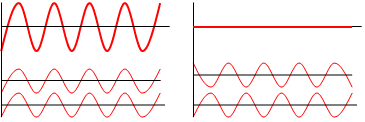# Standing waves

Hi all,actually i want to discuss about standing wave. Its little bit confusing to me.As a simple defintion,when two waves of equal amplitude travel in opposite direction then what the resultant wave,for which there is no propagation of energy is called standing waves.
But another property says that amplitude at each point in space stays constant and more the amplitude,more intense is the wave.Now question arises,If we are getting zero amplitude by superposition of 2 waves of equal amplitude,then what type of amplitude that is being discussed here. and we are saying that that all points have zero amplitude then how could we relate the intensity to amplitude.
thanks

Your question is a bit vague. If you are getting an amplitude of zero after superposition of two waves of equal amplitude, that means the two waves are exactly out of phase: the peaks cancel out the troughs exactly, like in the second (right-most) image here:If this is the case, there is no resultant sound wave and you cannot speak of its intensity.

jtbell
Mentor
There are two ways in which the word "amplitude" can be used in a standing wave. First, each point on the wave oscillates with some amplitude, which varies from one position to another along the wave. This amplitude is zero at a node and maximum at an antinode. Second, the standing wave as a whole has an amplitude which is the maximum amplitude of oscillation of any point on the wave, i.e. the amplitude at a node. [added: oops, I should have said "antinode" here.]

Last edited:
Hi jtbell,thanks for your response,but it is still confusing.On one side you said that amplitude is zero at a node and maximum at an antinode. On other side you said that ,the standing wave as a whole has an amplitude which is the maximum amplitude of oscillation, i.e. the amplitude at a node.
So kindly can you differentiate this.

I am pretty sure jtbell means the maximum amplitude of oscillation is at an antinode.

jtbell
Mentor
Oops yes, I meant the amplitude at an antinode.